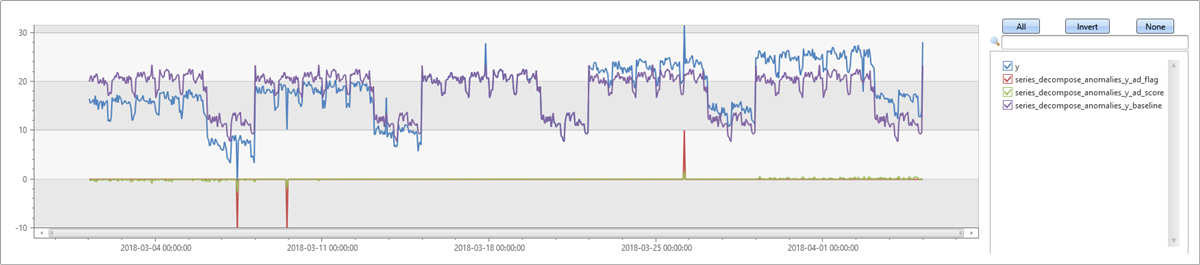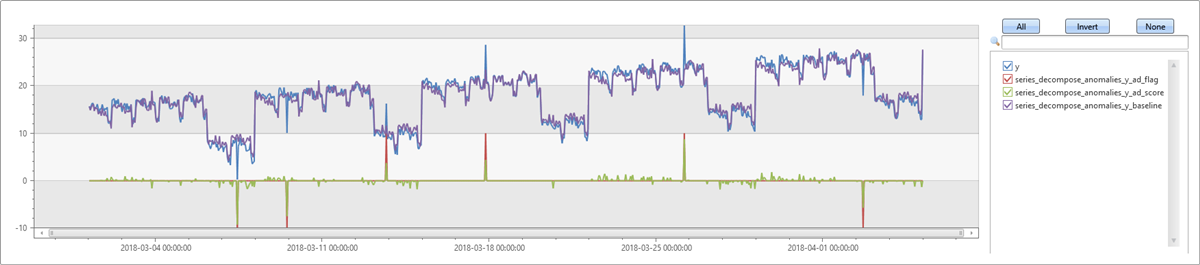# series_decompose_anomalies()

Anomaly Detection is based on series decomposition. For more information, see series_decompose().

The function takes an expression containing a series (dynamic numerical array) as input, and extracts anomalous points with scores.

## Syntax

`series_decompose_anomalies (`Series`,` [ Threshold`,` Seasonality`,` Trend`,` Test_points`,` AD_method`,` Seasonality_threshold ]`)`

## Parameters

Name Type Required Description
Series dynamic An array of numeric values, typically the resulting output of make-series or make_list operators.
Threshold real The anomaly threshold. The default is 1.5, k value, for detecting mild or stronger anomalies.
Seasonality int Controls the seasonal analysis. The possible values are:

- `-1`: Autodetect seasonality using series_periods_detect. This is the default value.
- Period: A positive integer specifying the expected period in number of bins. For example, if the series is in `1 - h` bins, a weekly period is 168 bins.
- `0`: No seasonality, so skip extracting this component.
Trend string Controls the trend analysis. The possible values are:

- `avg`: Define trend component as `average(x)`. This is the default.
- `linefit`: Extract trend component using linear regression.
- `none`: No trend, so skip extracting this component.
Test_points int A positive integer specifying the number of points at the end of the series to exclude from the learning, or regression, process. This parameter should be set for forecasting purposes. The default value is 0.
AD_method string Controls the anomaly detection method on the residual time series, containing one of the following values:

- `ctukey`: Tukey’s fence test with custom 10th-90th percentile range. This is the default.
- `tukey`: Tukey’s fence test with standard 25th-75th percentile range.

Seasonality_threshold real The threshold for seasonality score when Seasonality is set to autodetect. The default score threshold is 0.6.

## Returns

The function returns the following respective series:

• `ad_flag`: A ternary series containing (+1, -1, 0) marking up/down/no anomaly respectively
• `ad_score`: Anomaly score
• `baseline`: The predicted value of the series, according to the decomposition

## The algorithm

This function follows these steps:

1. Calls series_decompose() with the respective parameters, to create the baseline and residuals series.
2. Calculates ad_score series by applying series_outliers() with the chosen anomaly detection method on the residuals series.
3. Calculates the ad_flag series by applying the threshold on the ad_score to mark up/down/no anomaly respectively.

## Examples

### Detect anomalies in weekly seasonality

In the following example, generate a series with weekly seasonality, and then add some outliers to it. `series_decompose_anomalies` autodetects the seasonality and generates a baseline that captures the repetitive pattern. The outliers you added can be clearly spotted in the ad_score component.

``````let ts=range t from 1 to 24*7*5 step 1
| extend Timestamp = datetime(2018-03-01 05:00) + 1h * t
| extend y = 2*rand() + iff((t/24)%7>=5, 10.0, 15.0) - (((t%24)/10)*((t%24)/10)) // generate a series with weekly seasonality
| extend y=iff(t==150 or t==200 or t==780, y-8.0, y) // add some dip outliers
| extend y=iff(t==300 or t==400 or t==600, y+8.0, y) // add some spike outliers
| summarize Timestamp=make_list(Timestamp, 10000),y=make_list(y, 10000);
ts
| extend series_decompose_anomalies(y)
| render timechart
``````### Detect anomalies in weekly seasonality with trend

In this example, add a trend to the series from the previous example. First, run `series_decompose_anomalies` with the default parameters in which the trend `avg` default value only takes the average and doesn't compute the trend. The generated baseline doesn't contain the trend and is less exact, compared to the previous example. Consequently, some of the outliers you inserted in the data aren't detected because of the higher variance.

``````let ts=range t from 1 to 24*7*5 step 1
| extend Timestamp = datetime(2018-03-01 05:00) + 1h * t
| extend y = 2*rand() + iff((t/24)%7>=5, 5.0, 15.0) - (((t%24)/10)*((t%24)/10)) + t/72.0 // generate a series with weekly seasonality and ongoing trend
| extend y=iff(t==150 or t==200 or t==780, y-8.0, y) // add some dip outliers
| extend y=iff(t==300 or t==400 or t==600, y+8.0, y) // add some spike outliers
| summarize Timestamp=make_list(Timestamp, 10000),y=make_list(y, 10000);
ts
| extend series_decompose_anomalies(y)
series_multiply(10, series_decompose_anomalies_y_ad_flag) // multiply by 10 for visualization purposes
| render timechart
``````Next, run the same example, but since you're expecting a trend in the series, specify `linefit` in the trend parameter. You can see that the baseline is much closer to the input series. All the inserted outliers are detected, and also some false positives. See the next example on tweaking the threshold.

``````let ts=range t from 1 to 24*7*5 step 1
| extend Timestamp = datetime(2018-03-01 05:00) + 1h * t
| extend y = 2*rand() + iff((t/24)%7>=5, 5.0, 15.0) - (((t%24)/10)*((t%24)/10)) + t/72.0 // generate a series with weekly seasonality and ongoing trend
| extend y=iff(t==150 or t==200 or t==780, y-8.0, y) // add some dip outliers
| extend y=iff(t==300 or t==400 or t==600, y+8.0, y) // add some spike outliers
| summarize Timestamp=make_list(Timestamp, 10000),y=make_list(y, 10000);
ts
| extend series_decompose_anomalies(y, 1.5, -1, 'linefit')
series_multiply(10, series_decompose_anomalies_y_ad_flag) // multiply by 10 for visualization purposes
| render timechart
``````### Tweak the anomaly detection threshold

A few noisy points were detected as anomalies in the previous example. Now increase the anomaly detection threshold from a default of 1.5 to 2.5. Use this interpercentile range, so that only stronger anomalies are detected. Now, only the outliers you inserted in the data, will be detected.

``````let ts=range t from 1 to 24*7*5 step 1
| extend Timestamp = datetime(2018-03-01 05:00) + 1h * t
| extend y = 2*rand() + iff((t/24)%7>=5, 5.0, 15.0) - (((t%24)/10)*((t%24)/10)) + t/72.0 // generate a series with weekly seasonality and onlgoing trend
| extend y=iff(t==150 or t==200 or t==780, y-8.0, y) // add some dip outliers
| extend y=iff(t==300 or t==400 or t==600, y+8.0, y) // add some spike outliers
| summarize Timestamp=make_list(Timestamp, 10000),y=make_list(y, 10000);
ts
| extend series_decompose_anomalies(y, 2.5, -1, 'linefit')